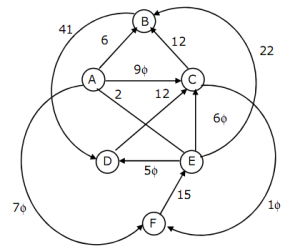# GATE | GATE CS 1996 | Question 65

Let G be the directed, weighted graph shown in below figureWe are interested in the shortest paths from A.

(a) Output the sequence of vertices identified by the Dijkstra’s algorithm for single source shortest path when the algorithm is started at node A.
(b) Write down sequence of vertices in the shortest path from A to E.
(c) What is the cost of the shortest path from A to E?

Explanation: According to Dijkstra’s algorithm:
(a) Sequence of vertices when algorithm start traversing from A: A -> B -> D -> C -> F -> E
(b) The sequence remain same as answer (a).
(c) Cost of the shortest path from A to E is 84.

Quiz of this Question

My Personal Notes arrow_drop_up

Improved By : arti111

Article Tags :

Be the First to upvote.

Please write to us at contribute@geeksforgeeks.org to report any issue with the above content.